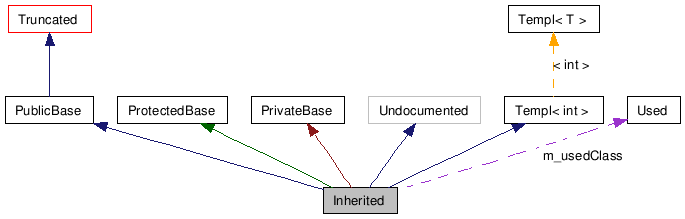# Graph Legend

This page explains how to interpret the graphs that are generated by doxygen.
Graph Graph Graph Graph Graph Graph Graph Graph Graph Graph Graph Graph Graph Graph Graph Graph Graph Graph Graph Graph Graph Graph Graph Graph Graph Graph Graph Graph Graph Graph Graph Graph Graph Graph Graph Graph Graph Graph Graph Graph Graph Graph Graph Graph Graph Graph Graph Graph Graph Graph Graph Graph Graph Graph Graph Graph Graph Graph Graph Graph Graph Graph Graph Graph Graph Graph Graph Graph Graph Graph Graph Graph Graph Graph Graph Graph Graph Graph Graph Graph Graph Graph Graph Graph Graph Graph Graph Graph Graph Graph Graph Graph Graph Graph Graph Graph Graph Graph Graph Graph Graph Graph Graph Graph Graph Graph Graph Graph Graph Graph Graph Graph Graph Graph Graph Graph Graph Graph Graph Graph
Consider the following example:
```/*! Invisible class because of truncation */
class Invisible { };

/*! Truncated class, inheritance relation is hidden */
class Truncated : public Invisible { };

/* Class not documented with doxygen comments */
class Undocumented { };

/*! Class that is inherited using public inheritance */
class PublicBase : public Truncated { };

/*! A template class */
template<class T> class Templ { };

/*! Class that is inherited using protected inheritance */
class ProtectedBase { };

/*! Class that is inherited using private inheritance */
class PrivateBase { };

/*! Class that is used by the Inherited class */
class Used { };

/*! Super class that inherits a number of other classes */
class Inherited : public PublicBase,
protected ProtectedBase,
private PrivateBase,
public Undocumented,
public Templ<int>
{
private:
Used *m_usedClass;
};
```
If the `MAX_DOT_GRAPH_HEIGHT` tag in the configuration file is set to 240 this will result in the following graph:The boxes in the above graph have the following meaning:

• A filled gray box represents the struct or class for which the graph is generated.
• A box with a black border denotes a documented struct or class.
• A box with a grey border denotes an undocumented struct or class.
• A box with a red border denotes a documented struct or class forwhich not all inheritance/containment relations are shown. A graph is truncated if it does not fit within the specified boundaries.
The arrows have the following meaning:
• A dark blue arrow is used to visualize a public inheritance relation between two classes.
• A dark green arrow is used for protected inheritance.
• A dark red arrow is used for private inheritance.
• A purple dashed arrow is used if a class is contained or used by another class. The arrow is labeled with the variable(s) through which the pointed class or struct is accessible.
• A yellow dashed arrow denotes a relation between a template instance and the template class it was instantiated from. The arrow is labeled with the template parameters of the instance.

Generated on Tue Feb 9 19:34:46 2010 for Python Reference Guide by1.5.3